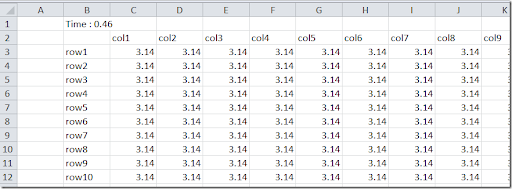## Thursday, December 5, 2013

### Populating a spreadsheet through VBA

Populating a large spreadsheet table can be slow if we push data cell-by-cell. Here is a small example.

##### Naïve approach

We start with creating a 1000x1000 table by directly accessing individual cells.

 Private Sub run1()    Dim r As Range    Set r = Range("Sheet1!B2")    r.Resize(m + 1, n + 1).ClearContents    Dim t0 As Single    t0 = Timer    Dim i As Integer    Dim j As Integer    For i = 1 To m       r(1 + i, 1) = "row" & i    Next i    For j = 1 To n       r(1, 1 + j) = "col" & j    Next j    For i = 1 To m       For j = 1 To n          r(1 + i, 1 + j) = 3.14       Next j    Next i    Dim t1 As Single    t1 = Timer    r(0, 1) = "Time : " & Format(t1 - t0, "fixed") End Sub

This approach takes about 240 seconds on my machine.

A refinement is to turn off screen updating: Application.ScreenUpdating = False. This did not make a difference for me. Excel seems to turn off screen updating automatically after a little while.

##### Fast approach

A better approach is to create a VBA array and to write this array in one swoop to the spreadsheet. We use here a Variant array as it contains both strings and numbers. The code looks like:

 Private Sub run3()    Dim r As Range    Set r = Range("Sheet3!B2")    r.Resize(m + 1, n + 1).ClearContents    Dim t0 As Single    t0 = Timer    Dim i As Integer    Dim j As Integer    Dim a(1 To m + 1, 1 To n + 1)    For i = 1 To m       a(1 + i, 1) = "row" & i    Next i    For j = 1 To n       a(1, 1 + j) = "col" & j    Next j    For i = 1 To m       For j = 1 To n          a(1 + i, 1 + j) = 3.14       Next j    Next i    r.Resize(m + 1, n + 1).Value = a    Dim t1 As Single    t1 = Timer    r(0, 1) = "Time : " & Format(t1 - t0, "fixed") End Sub

This code takes 0.46 seconds!This strategy is also useful when calling Excel from other languages such as Delphi or C#.